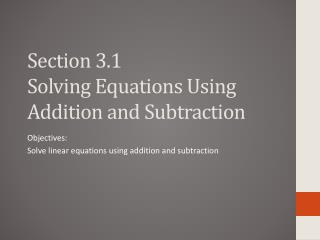# Section 3.1 Solving Equations Using Addition and Subtraction - PowerPoint PPT PresentationDownload PresentationSection 3.1 Solving Equations Using Addition and Subtraction

Section 3.1 Solving Equations Using Addition and SubtractionDownload Presentation## Section 3.1 Solving Equations Using Addition and Subtraction

- - - - - - - - - - - - - - - - - - - - - - - - - - - E N D - - - - - - - - - - - - - - - - - - - - - - - - - - -
##### Presentation Transcript

1. Section 3.1Solving Equations Using Addition and Subtraction Objectives: Solve linear equations using addition and subtraction

2. Addition and Subtraction Equations • You can solve an equation by using the transformations (inverse operations) to isolate the variable on one side of the equation. • Any transformations you apply to an equation must keep the equation in balance. Simplifying the sides of the equation to obtain the value of the variable. • LINEAR EQUATION: an equation in which the variable is raised to the first power and does not occur in a denominator, inside a square root symbol, or inside absolute value symbols. • INVERSE OPERATIONS: operations that undo each other

3. EX: Solve the equation • 1. 2. • 3. 4. • 5. 6.

4. EX: Match the real-life problem with an equation • a) b) c) • 1. You have x dollars and your friend repays you the \$7 he owes you. You now have \$9. How much did you have originally? • 2. A 9 foot post extends x feet below ground and 7 feet above the ground. What is the length x buried below ground? • 3. The temperature was x degrees F. It fell 2 degrees F and is now 7 degrees F. What was the original temperature?

5. Using Linear Equations in Real-life problems You are researching United States city parks. You note that Griffith Park is only 418 acres larger than Eagle Creek Park. Write an equation that models the size of Eagle Creek Park. Solve the equation. You note that the largest city park, Cullen Park, is only 248 acres smaller than the sum of the sizes of Griffith Park, Eagle Creek Park and Pelham Bay Park. Write an equation that models the size of Cullen Park. Solve the equation.

6. P. 13522 – 40 evens, 42 – 48 all, 57 – 75x3